# Properties of functions

## Increasing and decreasing functions

A function of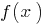increasing
if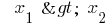,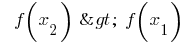for all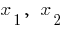of the region definitions (with the increase of the argument the corresponding points of the graph ascending stems)

The function ofthe descending
if,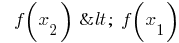for allof the region definitions (with the increase of the argument the corresponding points of the graph omitted)

## Even and odd functions

Functioneven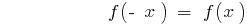for allof the region definitions. The graph of an even function is symmetrical to the axis vanocni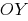A functionis odd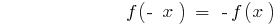for allof the region definitions. The graph of an odd function is symmetric vanocni the origin.

## The frequency

Definition: a Functionis called periodic with period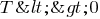if for anyof the determining function of the number and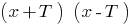also belong to the scope and the validity of the equation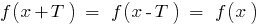.

Through the gaps in length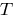of the graph of a periodic function repeats itself.

### Period features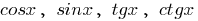Functions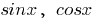have period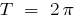.

Functions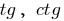have period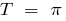.

Tags:
Chapter:
Versions in other languages:
Share with friends: